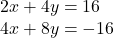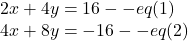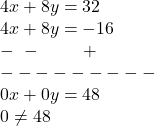2x + 4y = 16 4x + 8y = -16 he chooses to eliminate the variable y

Question

2x + 4y = 16
4x + 8y = -16 he chooses to eliminate the variable y

in progress 0
5 months 2021-08-15T04:12:53+00:00 1 Answers 6 views 0

These system of equations have no solution.

Step-by-step explanation:

We need to solve the system of equationsby eliminating variable y

Let:Multiply eq(1) by 2 and subtract both equationsAs 0≠48

So, these system of equations have no solution.